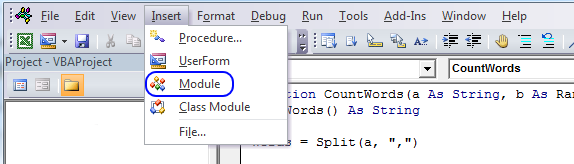Author: Oscar Cronquist Article last updated on October 09, 2018

I received an email from one of my seven blog readers.

In Excel, I have a column, say A, with some cells blank and some cells with text.

In another column, say B, each *cell* contains many words, separated by a comma.

For every cell in B, I need to check to see how many of the words from column A are in the cell and output the total count of matches.

I built a user defined function for this, if you have a regular formula you think can solve this, please share. This animated picture explains it all.

The formula in cell C2 is a user defined function. You build a udf just like a macro using the visual basic of application language (vba).

=CountWords(B2,\$A\$2:\$A\$20)

The first argument (B2) in this custom made function is a cell reference to comma-separated values, in a single cell. The second argument (\$A\$2:\$A\$20) is a reference to cell range that you want to count, make sure it is a single column cell reference.

### Build a user defined function

To build a user defined function, follow these steps:

1. Press Alt + F11 to open the visual basic editor
2. Click "Insert" on the menu
3. Click "Module"4. Copy the code below and paste it to the code module.

### User defined function vba code

```Function CountWords(a As String, b As Range)
Dim Words() As String
Dim Value As Variant, cell As Variant
Dim c As Single
Words = Split(a, ",")
For Each Value In Words
For Each cell In b
If UCase(WorksheetFunction.Trim(cell)) = UCase(WorksheetFunction.Trim(Value)) Then c = c + 1
Next cell
Next Value
CountWords = c
End Function
```

### Explaining the user defined function

Function name and arguments

A user defined function procedure always start with "Function" and then a name. This udf has two arguments, a and b. Variable a is a string and b is a range.

```Function CountWords(a As String, b As Range)
```

Declaring variables

```Dim Words() As String
Dim Value As Variant, cell As Variant
Dim c As Single
```

Words() is a dynamic string array.  Value and cell are variants. c is a single data type. Read more about Defining data types.

Split function

```Words = Split(a, ",")
```

The Split function accepts a text string and returns a zero-based, one-dimensional array containing all sub strings. Split allows you also to specify a delimiting character, default is the space character.

For ... Next statement

```For Each Value In Words
...
Next Value
```

Repeats a group of statements a specified number of times. In this case as many times as there are values in the array Words.

If function

```If UCase(WorksheetFunction.Trim(cell)) = UCase(WorksheetFunction.Trim(Value)) Then c = c + 1
```

The Ucase function converts a string to uppercase letters. The WorksheetFunction.Trim method removes all spaces from text except for single spaces between words.
The If function compares cell and Value and if they match 1 is added to c.

The udf returns...

```CountWords = c
```

The udf returns the value in c.

End a udf

```End Function
```

A function procedure ends with the "End function" statement.

### Download excel * .xlsm file

Count the number of cells within a range that meet multiple comma separated values.xlsm